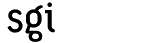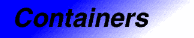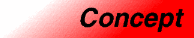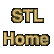# ContainerCategory: containers Component type: concept

### Description

A Container is an object that stores other objects (its elements), and that has methods for accessing its elements. In particular, every type that is a model of Container has an associated iterator type that can be used to iterate through the Container's elements.

There is no guarantee that the elements of a Container are stored in any definite order; the order might, in fact, be different upon each iteration through the Container. Nor is there a guarantee that more than one iterator into a Container may be active at any one time. (Specific types of Containers, such as Forward Container, do provide such guarantees.)

A Container "owns" its elements: the lifetime of an element stored in a container cannot exceed that of the Container itself. 

Assignable

### Associated types

 Value type X::value_type The type of the object stored in a container. The value type must be Assignable, but need not be DefaultConstructible.  Iterator type X::iterator The type of iterator used to iterate through a container's elements. The iterator's value type is expected to be the container's value type. A conversion from the iterator type to the const iterator type must exist. The iterator type must be an input iterator.  Const iterator type X::const_iterator A type of iterator that may be used to examine, but not to modify, a container's elements.   Reference type X::reference A type that behaves as a reference to the container's value type.  Const reference type X::const_reference A type that behaves as a const reference to the container's value type.  Pointer type X::pointer A type that behaves as a pointer to the container's value type.  Distance type X::difference_type A signed integral type used to represent the distance between two of the container's iterators. This type must be the same as the iterator's distance type.  Size type X::size_type An unsigned integral type that can represent any nonnegative value of the container's distance type. 

### Notation

 X A type that is a model of Container a, b Object of type X T The value type of X

### Definitions

The size of a container is the number of elements it contains. The size is a nonnegative number.

The area of a container is the total number of bytes that it occupies. More specifically, it is the sum of the elements' areas plus whatever overhead is associated with the container itself. If a container's value type T is a simple type (as opposed to a container type), then the container's area is bounded above by a constant times the container's size times sizeof(T). That is, if a is a container with a simple value type, then a's area is O(a.size()).

A variable sized container is one that provides methods for inserting and/or removing elements; its size may vary during a container's lifetime. A fixed size container is one where the size is constant throughout the container's lifetime. In some fixed-size container types, the size is determined at compile time.

### Valid expressions

In addition to the expressions defined in Assignable, EqualityComparable, and LessThanComparable, the following expressions must be valid.
Name Expression Type requirements Return type
Beginning of range a.begin()   iterator if a is mutable, const_iterator otherwise  
End of range a.end()   iterator if a is mutable, const_iterator otherwise 
Size a.size()   size_type
Maximum size a.max_size()   size_type
Empty container a.empty()   Convertible to bool
Swap a.swap(b)   void

### Expression semantics

Semantics of an expression is defined only where it differs from, or is not defined in, Assignable, Equality Comparable, or LessThan Comparable
Name Expression Precondition Semantics Postcondition
Copy constructor X(a)     X().size() == a.size(). X() contains a copy of each of a's elements.
Copy constructor X b(a);     b.size() == a.size(). b contains a copy of each of a's elements.
Assignment operator b = a     b.size() == a.size(). b contains a copy of each of a's elements.
Destructor a.~X()   Each of a's elements is destroyed, and memory allocated for them (if any) is deallocated.
Beginning of range a.begin()   Returns an iterator pointing to the first element in the container.  a.begin() is either dereferenceable or past-the-end. It is past-the-end if and only if a.size() == 0.
End of range a.end()   Returns an iterator pointing one past the last element in the container. a.end() is past-the-end.
Size a.size()   Returns the size of the container, that is, its number of elements.  a.size() >= 0 && a.size() <= max_size()
Maximum size a.max_size()   Returns the largest size that this container can ever have.  a.max_size() >= 0 && a.max_size() >= a.size()
Empty container a.empty()   Equivalent to a.size() == 0. (But possibly faster.)
Swap a.swap(b)   Equivalent to swap(a,b) 

### Complexity guarantees

The copy constructor, the assignment operator, and the destructor are linear in the container's size.

begin() and end() are amortized constant time.

size() is linear in the container's size.  max_size() and empty() are amortized constant time. If you are testing whether a container is empty, you should always write c.empty() instead of c.size() == 0. The two expressions are equivalent, but the former may be much faster.

swap() is amortized constant time. 

### Invariants

 Valid range For any container a, [a.begin(), a.end()) is a valid range.  Range size a.size() is equal to the distance from a.begin() to a.end(). Completeness An algorithm that iterates through the range [a.begin(), a.end()) will pass through every element of a. 

### Notes

 The fact that the lifetime of elements cannot exceed that of of their container may seem like a severe restriction. In fact, though, it is not. Note that pointers and iterators are objects; like any other objects, they may be stored in a container. The container, in that case, "owns" the pointers themselves, but not the objects that they point to.

 This expression must be a typedef, that is, a synonym for a type that already has some other name.

 This may either be a typedef for some other type, or else a unique type that is defined as a nested class within the class X.

 A container's iterator type and const iterator type may be the same: there is no guarantee that every container must have an associated mutable iterator type. For example, set and hash_set define iterator and const_iterator to be the same type.

 It is required that the reference type has the same semantics as an ordinary C++ reference, but it need not actually be an ordinary C++ reference. Some implementations, for example, might provide additional reference types to support non-standard memory models. Note, however, that "smart references" (user-defined reference types that provide additional functionality) are not a viable option. It is impossible for a user-defined type to have the same semantics as C++ references, because the C++ language does not support redefining the member access operator (operator.).

 As in the case of references , the pointer type must have the same semantics as C++ pointers but need not actually be a C++ pointer. "Smart pointers," however, unlike "smart references", are possible. This is because it is possible for user-defined types to define the dereference operator and the pointer member access operator, operator* and operator->.

 The iterator type need only be an input iterator, which provides a very weak set of guarantees; in particular, all algorithms on input iterators must be "single pass". It follows that only a single iterator into a container may be active at any one time. This restriction is removed in Forward Container.

 In the case of a fixed-size container, size() == max_size().

 For any Assignable type, swap can be defined in terms of assignment. This requires three assignments, each of which, for a container type, is linear in the container's size. In a sense, then, a.swap(b) is redundant. It exists solely for the sake of efficiency: for many containers, such as vector and list, it is possible to implement swap such that its run-time complexity is constant rather than linear. If this is possible for some container type X, then the template specialization swap(X&, X&) can simply be written in terms of X::swap(X&). The implication of this is that X::swap(X&) should only be defined if there exists such a constant-time implementation. Not every container class X need have such a member function, but if the member function exists at all then it is guaranteed to be amortized constant time.

 For many containers, such as vector and deque, size is O(1). This satisfies the requirement that it be O(N).

 Although [a.begin(), a.end()) must be a valid range, and must include every element in the container, the order in which the elements appear in that range is unspecified. If you iterate through a container twice, it is not guaranteed that the order will be the same both times. This restriction is removed in Forward Container.